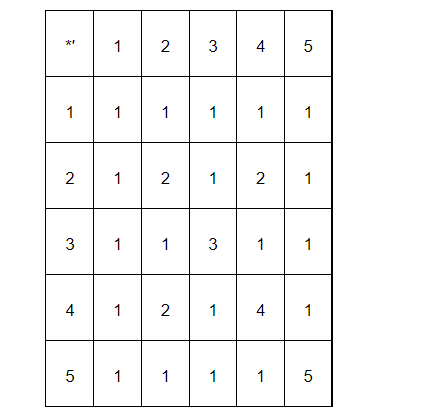# Let*′ be the binary operation on the set {1, 2, 3, 4, 5} defined by a *′ b = H.C.F.

Question:

Let ${ }^{* \prime}$ be the binary operation on the set $\{1,2,3,4,5\}$ defined by $a^{* \prime} b=$ H.C.F. of $a$ and $b$. Is the operation $^{* \prime}$ same as the operation * defined in Exercise 4 above? Justify your answer.

Solution:

The binary operation *' on the set $\{1,2,34,5\}$ is defined as $a^{* \prime} b=$ H.C.F of $a$ and $b$.

The operation table for the operation ${ }^{* \prime}$ can be given as:We observe that the operation tables for the operations ${ }^{*}$ and ${ }^{* \prime}$ are the same.

Thus, the operation *' is same as the operation*